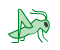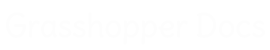﻿ Tween Through Vectors - Pufferfish - Component for Grasshopper | Grasshopper DocsCommunity documentation for Grasshopper add-ons & pluginsColor
 Curve
 Domain
 List
 Mesh
 Number
 Plane
 Point
 SubD
 Surface
 Transform
 Twisted Box
 Vector

# Tween Through Vectors

## Inputs

NameIDDescriptionType
VectorsVSet of vectors to tween throughVector
FactorFTween factor (0.0 = Vector at V start, 1.0 = Vector at V end), unless Normalized is FalseNumber
NormalizedNIf False, the rules for factor are (0.0 = Vector at index 0, Index number of last vector = Vector at last index) The tween factor range from one vector to the next vector in the list is: Index number of a vector = the vector at that index, Index number of the next vector = the next vector in the list, factors between the vector index numbers will be the corresponding tweens. For instance: A tween factor of 2.5 will result in a tween vector halfway between the vector at index 2 and the vector at index 3.Boolean
EqualizedEIf True, tween distribution is evaluated along the tween path without considering the locations of the input vector setBoolean
WeightedWIf True, tween distribution is weighted by the input vector set and the tween path curvatureBoolean
LoopbackLIf True, allow the tween path to continue from the end back to the start as a closed loop (if Normalized is False, increase the tween factor range maximum by 1)Boolean
DegreeDCurvature degree of the tween path, degree should be a positive odd numberInteger
Interpolation TypeIDetermines how the tween path is interpolated 0 = Linear 1 = Chord 2 = Square Root 3 = UniformInteger

## Outputs

NameIDDescriptionType
TweenTResulting tween vectorVector

## Video Tutorials

Site design © Robin Rodricks.   Pufferfish and associated data © 2023 Michael Pryor.
Rhinoceros and Grasshopper are registered trademarks of Robert McNeel & Associates.  Hosted by GitHub